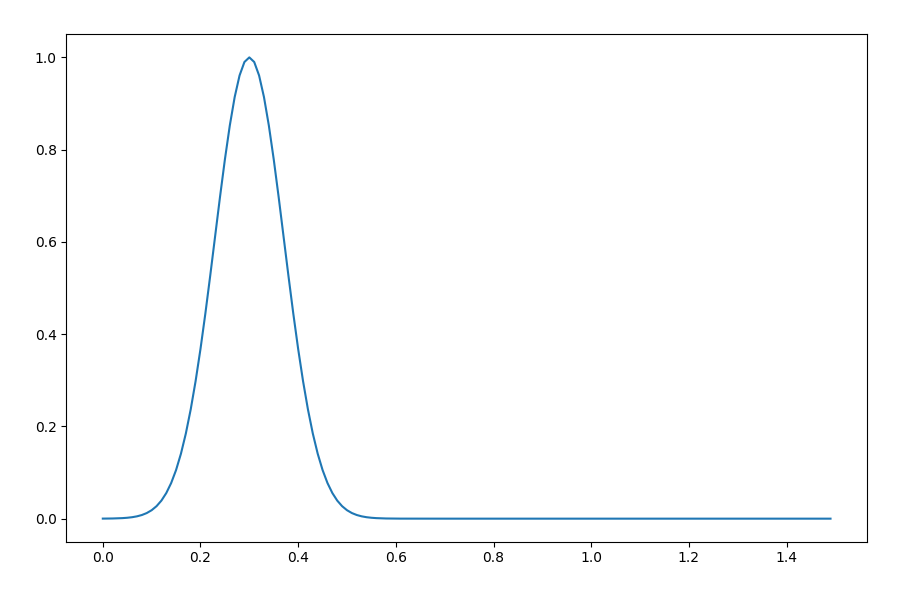# Testing¶

Testing and/or debugging the logic implemented in process classes can be achieved easily just by instantiating them. The xarray-simlab framework is not invasive and process classes can be used like other, regular Python classes.

Here is an example with the InitUGauss process class created in Section Create and Modify Models:

In : import numpy as np

In : import matplotlib.pyplot as plt

In : gauss = InitUGauss(loc=0.3, scale=0.1, x=np.arange(0, 1.5, 0.01))

In : gauss.initialize()

In : plt.plot(gauss.x, gauss.u);Like for any other process class, the parameters of the InitUGauss constructor correspond to each of the variables declared in that class with either intent='in' or intent='inout'. Those parameters are “keyword only” (see PEP 3102), i.e., it is not possible to set these as positional arguments.